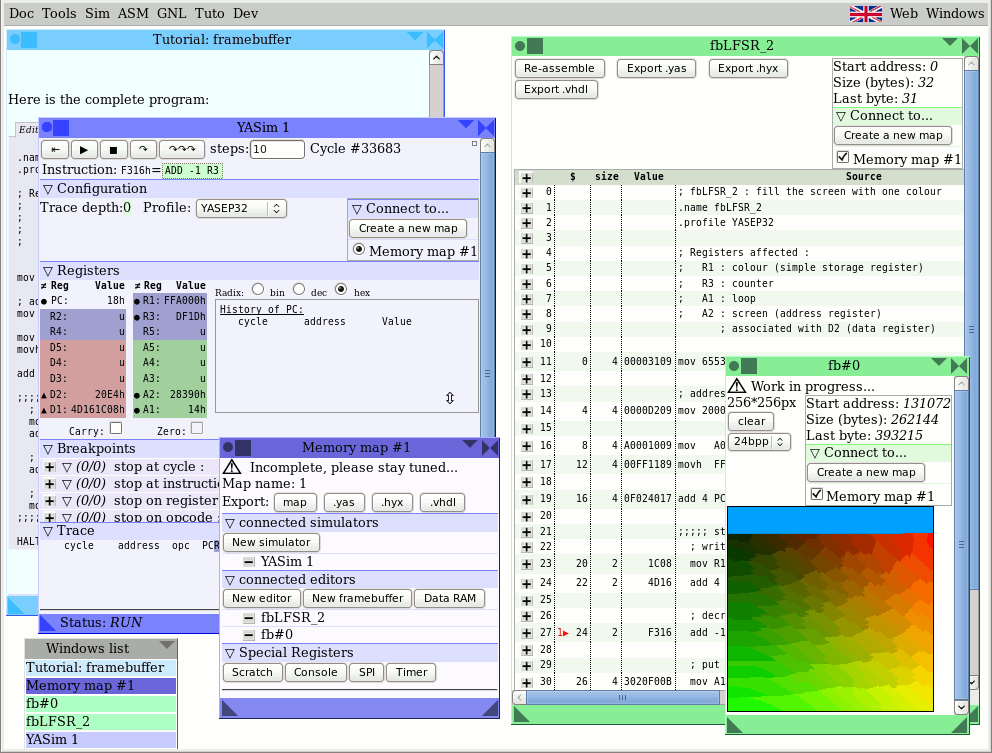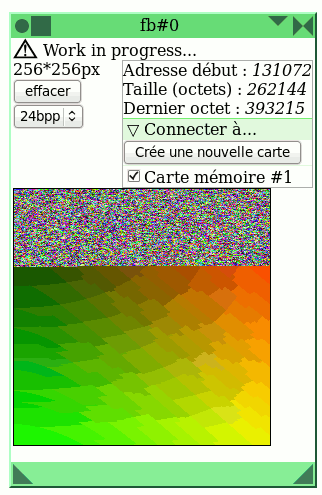Tutorial: framebuffer
yasep/tuto/tuto_fb created 2012-11-18 by Laura Bécognée & Yann Guidon
version 2012-11-23
version 2013-08-04 : translation contributed by Jeff Framebugger Davies

Introduction to graphics programming with the YASEP

Introduction

This small tutorial demonstrates graphics programming by writing a little program for the YASEP, step by step, with a simple example : filling the framebuffer with a pseudo random colour.

Requirements and Assumptions:

• You know how to use the Web-IDE interface, editing a program and running a simulation.
• The processor is the YASEP32 - the addresses and data are 32 bits wide.
• The program starts by default at address 0.
• The framebuffer is positioned at an arbritrary address 0x20000, consists of 256×256 pixels and each pixel is a 32 bit word (one processor word) with 24 bits being the colour (using mode truecolour "24bpp") making a total of 256Ki bytes.

⚠ The code doesn't work if the framebuffer window is not open. ⚠

Don't forget to open it (click here) and make sure it is linked to the same memory map as the editors.

Programming a loop.

To fill the screen you have to address all of the pixels using a loop.

Start by assigning registers for this operation:

```;  R3 : a counter
;  A1 : stores the return address for the loop
; Note : this is a comment. Not necessary,
; but useful for navigating the program.
```

Then we must fill register R3 with the right number of iterations. The YASEP is configured as 32 bits and the framebuffer uses 24 bits per pixel (with 8 most significant bits unused), therefore it's possible to access 1 pixel at a time.

With a framebuffer of 256*256, that makes 65,636 pixels and therefore there must be 65536 iterations to fill the whole frame buffer. The value is decremented each pass.

`  mov 65536 R3`

We must also create an instruction in order to save the loop address to allow the loop to return to the right place in the program.

We calculate 4+PC and put the result in A1. The PC register contains the address of the current instruction. We create a pointer to the following instruction (PC+4) so that later we can jump back to the right place in the loop. We add 4 because this instruction word is 4 bytes long, but beware there are also 2 byte instructions, so read the documentation for the instructions you're using.

`  add 4 PC A1`

And then decrement the counter.

`  add -1 R3`

Then copy A1 into the PC (Program Counter) if R3 is not zero (NZ).

`  mov A1 PC NZ R3`

And finally halt the CPU when the program is complete.

`  HALT 1`

Here is the complete program: :

```; fbLFSR_1 : loop 65536 times
.name fbLFSR_1
.profile YASEP32

; Registers affected :
;   R3 : counter
;   A1 : loop

mov 65536 R3

;;;;; start of the loop ;;;;;
; decrement the counter

; move A1 into the PC if R3 is not equal to 0
mov A1 PC NZ R3
;;;;;; end of the loop ;;;;;;

HALT 1```

Changing the colour of the framebuffer

Now, we access the framebuffer, by calculating the address of the pixels so we can change their value (to a single colour).

We start by assigning the registers:

```;  R1 : colour (R : simple storage register)
;  R3 : counter
;  A1 : loop
;  A2 : screen (A : address register)
;       associated with D2 (D : data register)```

We now point A2 to the beginning of the framebuffer:

`  mov 20000h A2`

The framebuffer address could change arbitrarily, check that this corresponds with the address defined at the top right of the framebuffer window.

Then decide the colour of the pixels:

For this, you have to place #00A0FF (the HTML code of the colour) in R1. We start by restructuring the byte order in the word:

• 00 : for the colour red
• A0 : for the colour green
• FF : for the colour blue

We manually convert the RGB colour into BGR because the YASEP is "Little Endian" (the lower byte of a register goes to the first address) and the red component is the first byte position in the pixel word. The colour value will ultimately be 00 A0 FF 00 (the first two 0 are not used but they are required to fill a 32 bits word).

The YASEP cannot load 32 bits of immediate data at once, it's therefore necessary to have a sequence of instructions that change each of the 16 bit half words at a time. First the value A0 00 is placed in the rightmost significant 16 bits with MOV then (00) FF in the left most significant 16 bits using MOVH..

```  mov   A000h R1
movh  FFh   R1 ; #00A0FF : sky blue```

Then we write the color in the framebuffer with MOV and advance to the next pixel (4 bytes later) with ADD 4 A2:

```  mov R1 D2
```

Here is the complete program:

```; fbLFSR_2 : fill the screen with one colour
.name fbLFSR_2
.profile YASEP32

; Registers affected :
;   R1 : colour (simple storage register)
;   R3 : counter
;   A1 : loop
;   A2 : screen (address register)
; associated with D2 (data register)

mov 65536 R3

mov 20000h A2

mov   A000h R1
movh  FFh   R1 ; #00A0FF : sky blue

;;;;; start of the loop ;;;;;
; write the colour in the framebuffer
mov R1 D2

; decrement the counter

; put the contents of A1 into the PC if R3 is not equal to 0
mov A1 PC NZ R3
;;;;;; end of loop ;;;;;;

HALT 1```

This is what your screen should look like:Changing the colour with a LFSR.

We are now going to fill the screen with pseudo-random coloured pixels. To do this, we use a "Linear FeedBack Shift Register". The math theory is well described on the English wikipedia page and a very detailed article on it is available in french on the website UnixGarden. The principle is reduced to one shift operation and one XOR (exclusive-or) operation.

All of the subtleties of the maths are reduced to the constant chosen for the XOR operation, which is called a "polynomial". There are tables of valid 32 bit values that you can choose, and the 32 bit one used here comes from the CRC32 standard : 0x04C11DB7

For the pseudo-random number generator to function correctly, in addition to the choice of a working polynomial, you must also make sure that R1 is not initialized with the value 0. The colour "sky blue" is a convenient example of a non-zero value that R1 can be initialised with.

Registers Affected:

```;   R1 : LFSR (colour)
;   R2 : polynomial
;   R3 : counter
;   A1 : loop
;   A2 : screen (Address Register)
;        associated with D2 (Data register)```

We therefore initialize the LFSR's polynomial in R2.

```  mov   1DB7h R2 ; LSB
movh 04C1h R2 ; MSB```

Like the blue sky colour, this is a value that doesn't fit in 20 bits, so it must be "cut in half" then loaded into R2 using a sequence of two instructions (MOV then MOVH).

... and then we generate a pseudo-random bit ::

```  add R1 R1 ; shift the register to the left by one bit
; and copy the most significant bit's value into the carry bit```

Finally, if the Carry flag (coming from R1's most significant bit) is 1, XOR the result with the polynomial. This allows us to generate the pseudo-random bit in combination with the offset produced by the previous ADD:

`  xor R2 R1 CARRY`

Here is the complete program:

```; fbLFSR : fill the screen with
; a pseudo-random colour
.name fbLFSR
.profile YASEP32

; Registers affected :
;   R1 : LFSR (colour)
;   R2 : polynomial
;   R3 : counter
;   A1 : loop
;   A2 : screen (address register)
; associated with D2 (data register)

mov 65536 R3

mov 20000h A2

mov   A000h R1
movh  FFh   R1 ; #00A0FF : sky blue

; initialise R2 with the LFSR polynomial:
mov  1DB7h R2 ; LSB
movh 04C1h R2 ; MSB

;;;;; start of the loop ;;;;;
; generate a pseudo-random bit :
ADD R1 R1 ; shift R1 by one position
; to the left and copy the most
; significant bit into the carry flag

; If the carry flag is set, apply the polynomial
xor R2 R1 CARRY

; write the colour into the framebuffer
mov R1 D2

; decrement the counter